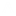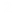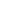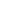1 {-
2 From "Pearls of Functional Algorithm Design", by Richard Bird
3
4 Chapter 9.
5
6 ** Problem: Celebrity Clique.
7
8 Set of P people in a Party.
9 Subset C of P is called "Celebrity Clique" if it's not empty,
10 and everyone in the party knows every member of C, but members
11 C know only each other.
12
13 Assumin gthere is such a clique, we need to find it.
14
15 We are given the set P (list, not containing duplicates),
16 and a binary predicate 'knows'.
17
18 ** Formaulation of the problem
19 Set annotation:
20 C is celebrity-clique of P if
21 C \in P, C \not empty , and
22 { \forevery x \in P, \forevery y \in C ::
23             x knows y  AND ( y knows x ==> x \in C )}
24
25 ** Solution (Brute force):
26     Create all subsequences.
27     For each subsequence, check if the condition above holds.
28
29 ** Solution (in Linear time)
30     See the book for full explanation. The point: We know there IS a clique.
31     we just need to find it.
32 -}
33
34 module P9 where
35 -- For our party, we will have a set of 6 people.
36 -- The celebrity clique will be 1 and 3.
37 ps = [1..6] :: [Int]
38 aKnowsb :: [(Int,Int)]
39 aKnowsb =   [ (2,1),(3,1),(4,1),(5,1),(6,1)] ++
40             [ (1,3),(2,3),(4,3),(5,3),(6,3)] ++
41             [ (2,4),(5,4)]
42
43 knows :: Int -> Int -> Bool
44 knows x y = (x,y) elem aKnowsb
45
46 --------------------
47 --  Brute force solution
48 cclique :: [Int] -> [Int]
49 cclique ps = head (filter (\x -> isCC x ps) (subseqs ps))
50
51 -- is this subgroup a celebrity-clique?
52 isCC :: [Int] -> [Int] -> Bool
53 isCC cc ps = and [  (x knows y)  &&
54                 ( (not (y knows x) ) || ((y knows x) && (x elem cc)) )
55                 | x<-ps , y<-cc, x /=y]
56
57 -- All possible subsequences, in descending order of length
58 -- (longest one first)
59 subseqs :: [Int] -> [[Int]]
60 subseqs []      = [[]]
61 subseqs (x:xs)  = map (x:) (subseqs xs) ++ subseqs xs
62
63
64 --------------------
65 --  Linear solution
66
67 -- just noting. This is the base for the fusion work.
68 -- Not used here, but used in the derivation.
69 subseqs' :: [Int] -> [[Int]]
70 subseqs' xs = foldr add [[]] xs
71
72 add :: Int -> [[Int]] -> [[Int]]
73 add x xss = map (x:) xss ++ xss
74
75 cclique' :: [Int] -> [Int]
76 cclique' ps = foldr op [] ps
77
78 -- cs is the current clique. p is the new person
79 op :: Int -> [Int] -> [Int]
80 op p cs |   null cs             = [p]   -- We know there's a clique, so if it's
81                                         -- empty so far, p is 'it'.
82         |   not (p knows c)   = [p]   -- if the new person doesn't know c,
83                                         -- cs is blown off, and we start afresh.
84         |   not (c knows p)   = cs    -- if we got here, that means that p knows c
85                                         -- now, if c doesn't know p, we are good with cs
86                                         -- as is!
87         |   otherwise           = p:cs  -- and if c does know p, then p is part
88                                         -- of the clique.
89         where
99     print $cclique' ps 100 putStr "Linear time method : " 101 print$ cclique' ps